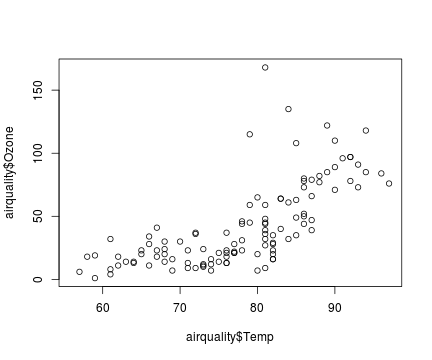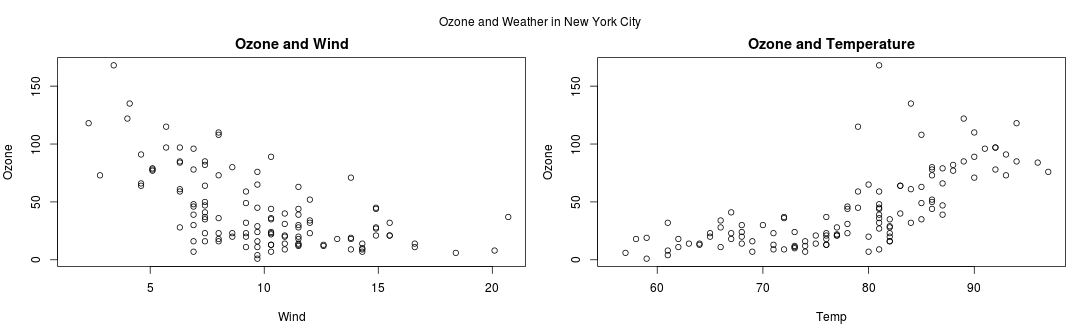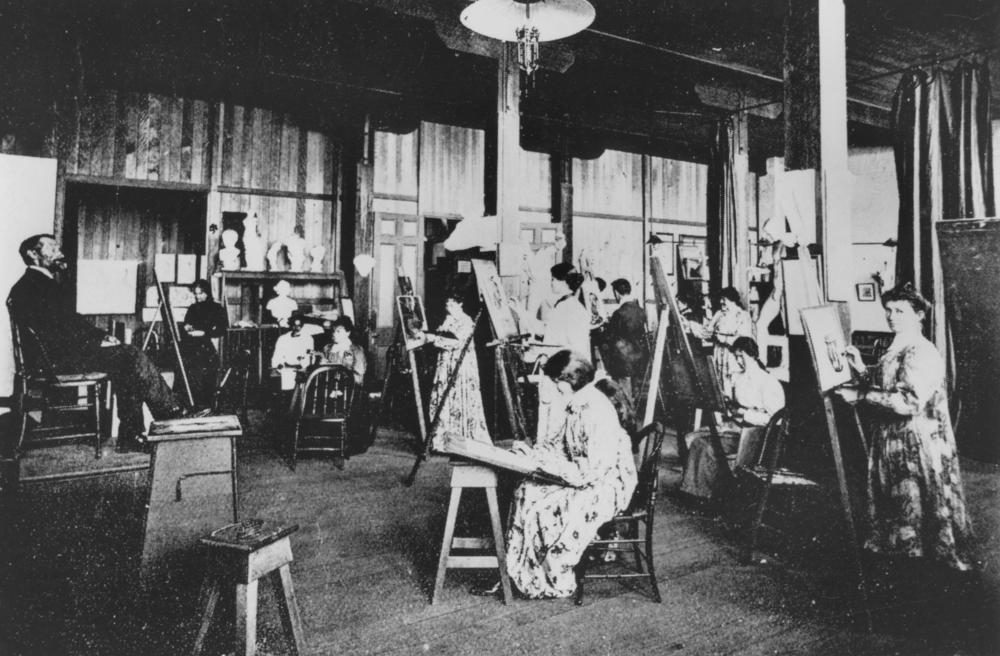# Basic plotting systems

1. Base graphics: constructed piecemeal. Conceptually simpler and allows plotting to mirror the thought process.
2. Lattice graphics: entire plots created in a simple function call.
3. ggplot2 graphics: an implementation of the Grammar of Graphics by Leland Wikinson. Combines concepts from both base and lattice graphics. (Need to install ggplot2 library)
4. Fancier and more telling ones.

A list of interactive visualization in R can be found at: http://ouzor.github.io/blog/2014/11/21/interactive-visualizations.html

## Base plotting system

``````library(datasets)
## scatter plot
plot(x = airquality\$Temp, y = airquality\$Ozone)
``````## Base plotting system

``````## par() function is used to specify global graphics parameters that affect all plots in an R session.
## Type ?par to see all parameters
par(mfrow = c(1, 2), mar = c(4, 4, 2, 1), oma = c(0, 0, 2, 0))
with(airquality, {
plot(Wind, Ozone, main="Ozone and Wind")
plot(Temp, Ozone, main="Ozone and Temperature")
mtext("Ozone and Weather in New York City", outer=TRUE)})
``````## Plotting functions (high level)PHASE ONE: Mount a canvas panel on the easel, and draw the draft. (Initialize a plot.)

• plot(): one of the most frequently used plotting functions in R.
• boxplot(): a boxplot show the distribution of a vector. It is very useful to example the distribution of different variables.
• barplot(): create a bar plot with vertical or horizontal bars.
• hist(): compute a histogram of the given data values.
• pie(): draw a pie chart.

Remember to use ?plot or str(plot), etc. to check the arguments when you want to make more personalized plots. A tutorial of base plotting system with more details: http://bcb.dfci.harvard.edu/~aedin/courses/BiocDec2011/2.Plotting.pdf